Help Center
1. Help Center
2. Working in the Platform
3. Basic Elements of Projects

# Variable Rates

## Use variable pricing to setup pricing structures that changes based on quantity.

### Variable Pricing gives you more control over how to price projects.

Using variable pricing parameters, you can manipulate the level of effort, service price, service cost, product price, and product cost based on the quantity of the instance of a service or product in a project.

### Configuring Variable Rates in Settings

To use variable rates, first set up Service or Product in Settings.

• For Professional Services, you can set up hours or Service Price/Cost-based variable rates.

• For Managed Services, you will only have the option to setup Service Price/Cost-based variable rates.

• For Products, you can set up Product Price/Cost variable rates.

In the below example, we will look at setting up a service with variable rates and will explain the function of all the types of variable rates as we go.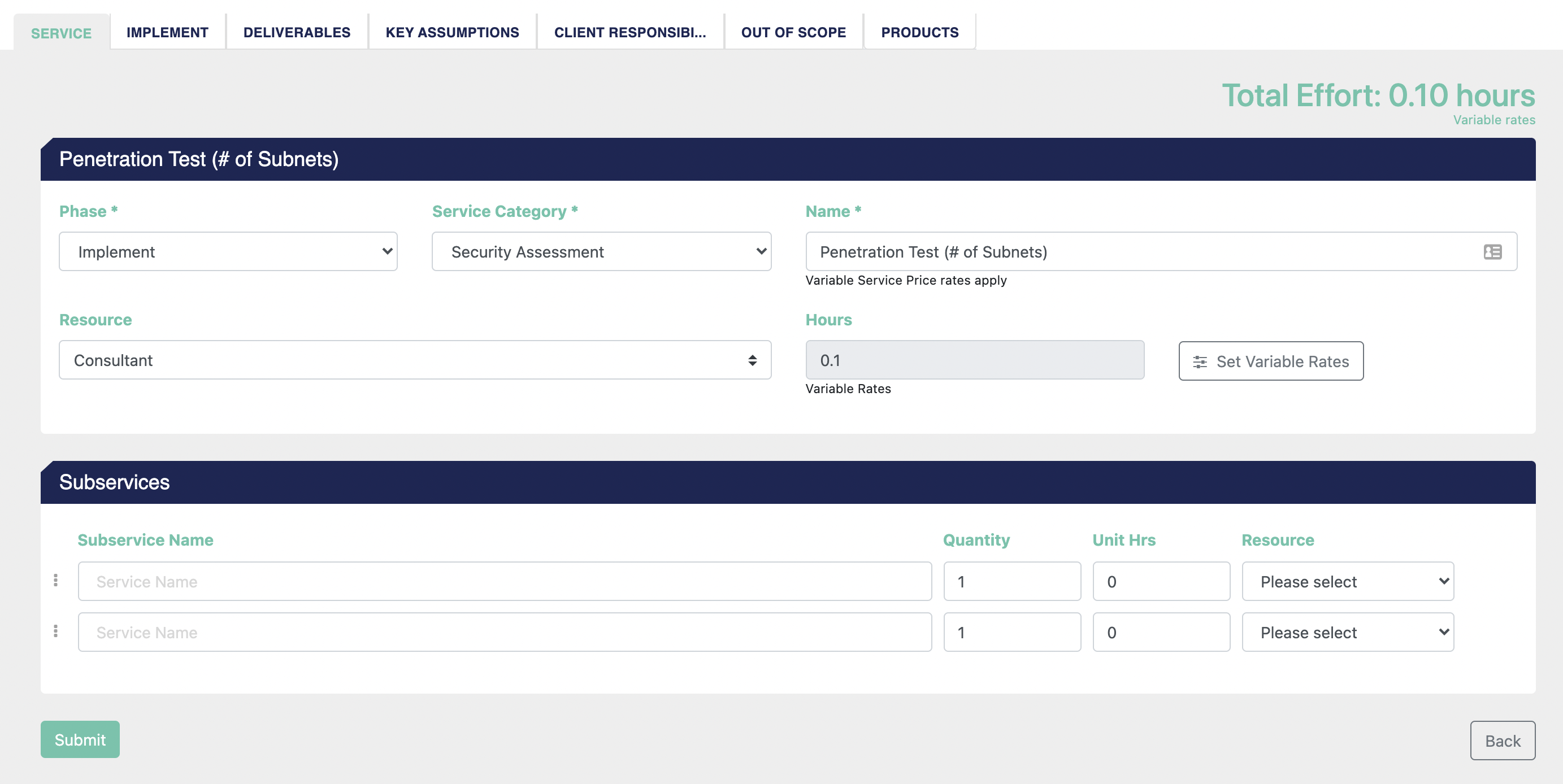Once you have created the Service and saved it, you will see the option to Set Variable Rates, available as a button in the top-level portion of the service, and in the drop-down context menu on each subservice.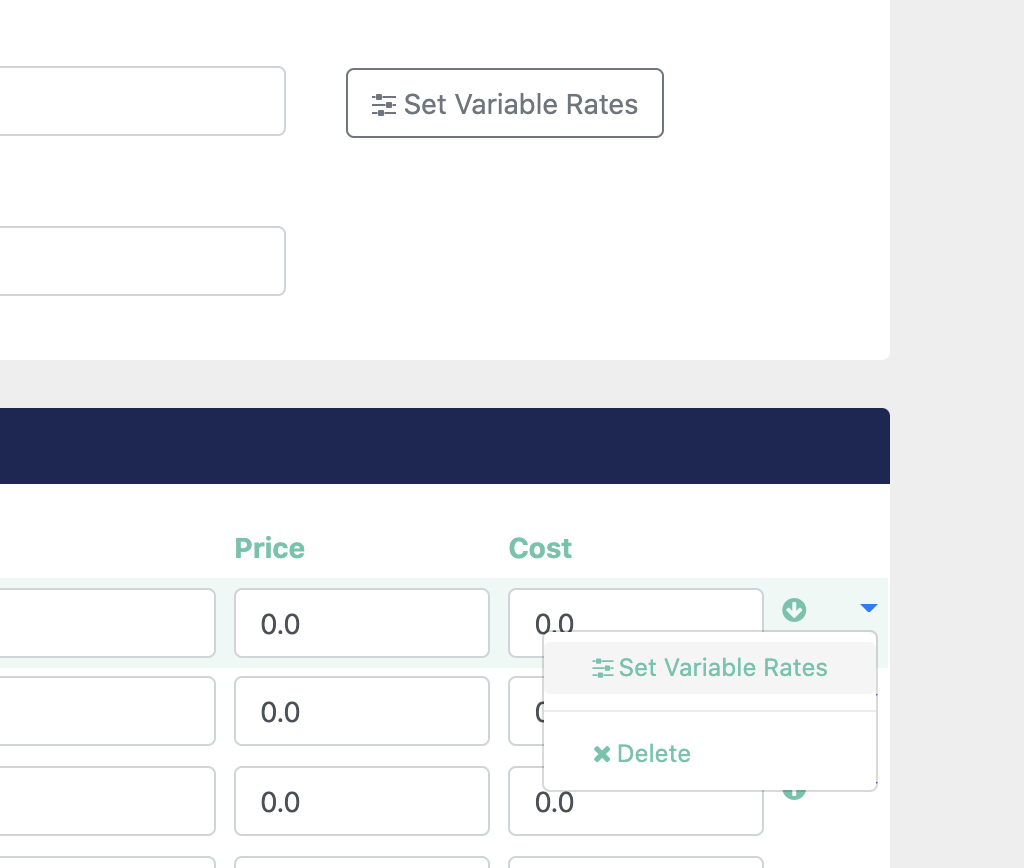Users can set independent variable rates on each element of the service and subservice using this user interface: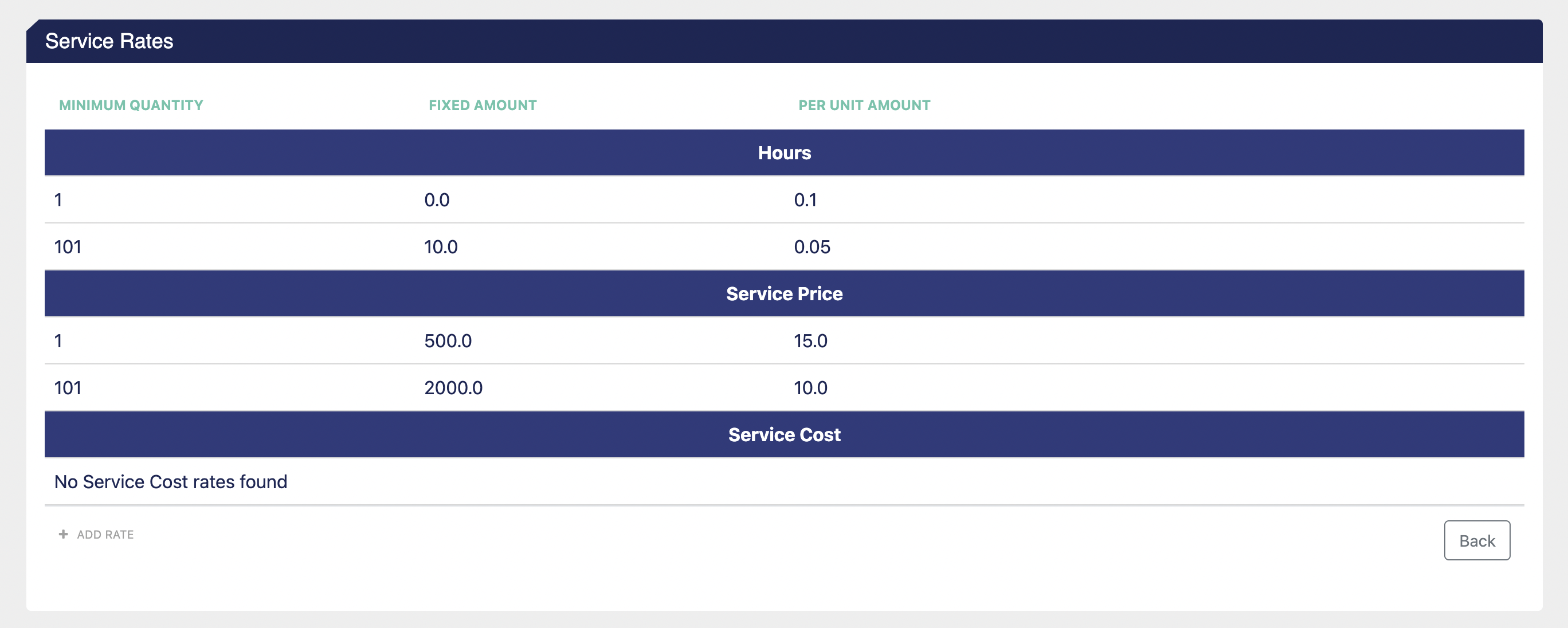Note that our example above is for a Service, so Product Price & Cost are not available, but those functions are available on a Product.

The areas of the service you can set a variable rate on are:

• Hours 🕑: You can configure the platform to calculate the level of effort needed to complete the service, based on the quantity tiers of a specific service or subservice. Units in this area are hours.

• Service Price 💵: You can configure the platform to override the Resource Rate and level of effort to achieve a fixed fee price for the service or subservice based on different quantity levels that you define. Units in this area are dollars.

• Service Cost 💵: You can configure the platform to override the Resource Cost and calculate the cost of the service or subservice based on different quantity levels that you can define here. Units in this area are dollars.

• Product Price 💵: You can instruct the platform to calculate the Product Price based on different quantity levels that you can define here. Units in this area are dollars.

• Product Cost 💵: You can instruct the platform to calculate the Product Cost based on different quantity levels that you can define here. Units in this area are dollars.

The individual elements you can define per minimum quantity are:

• Fixed Amount: This is the amount to account for quantities below the minimum quantity.

• Per Unit Amount: This is the amount added per unit at and above the minimum quantity threshold you define, up until (but not including) the next minimum quantity, if applicable.

### 🕒 Hours-based Variable Rates

For this Variable Rates structure for hours on a service or subservice: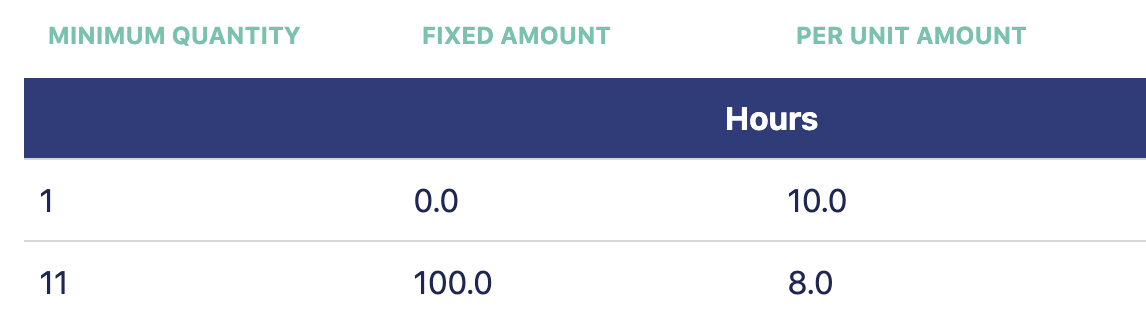When I add this service to a project, for quantities 1-10, it will only add 10 hours for every unit of quantity I add. There are 0 fixed hours, so no amount is added there.

• For quantity = 1, effort is 10 hours (0 fixed + 10 per unit * 1 quantity)

• For quantity = 5, effort is 50 hours (0 + 10*5)

• For quantity = 10, effort is 100 hours (0 + 10*10)

For quantities of 11 and up, there is a base of 100 hours added initially, and then 8 hours added for every additional increment.

• For quantity = 11, effort is 108 hours (100 + 8*1)

• For quantity = 15, effort is 140 hours (100 + 8*5)

• For quantity = 100, effort is 820 hours (100 + 8*90)

### 💵 Dollars-based Variable Rates

The same logic applies if you set up a Variable Rate service for service price/cost or product price/cost.

For this Variable Rates structure for the Hours & Service Price on a service or subservice: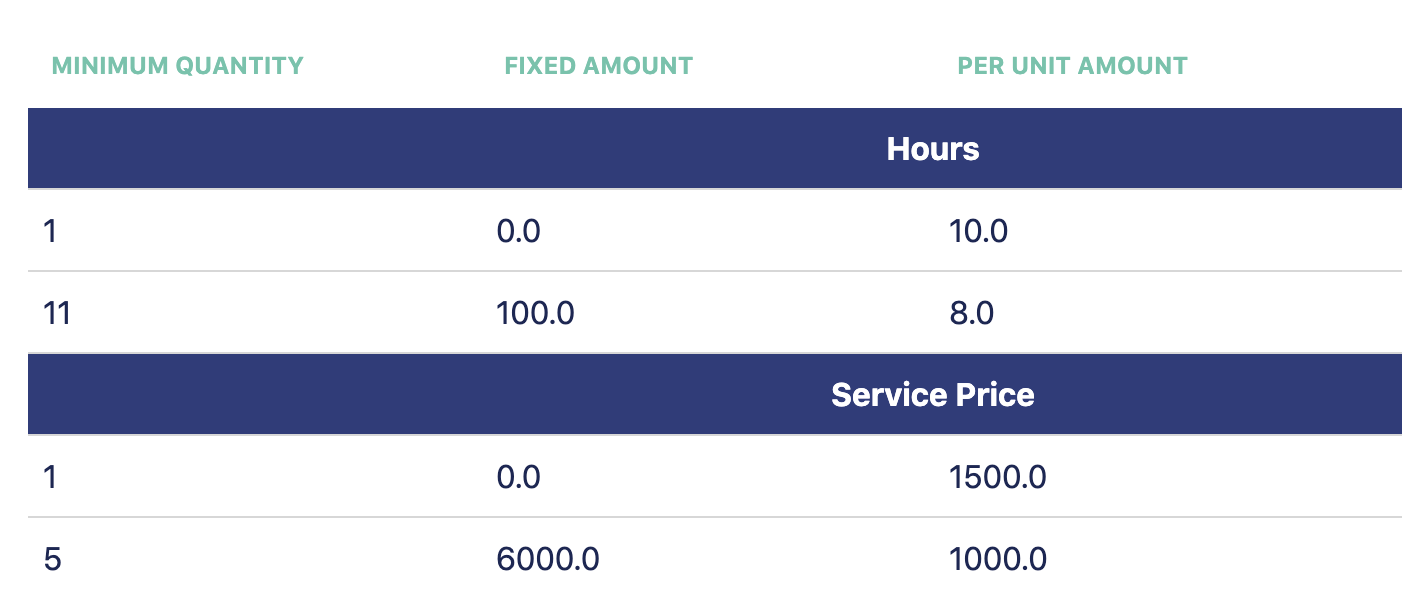Since I have not defined a Variable Rate for Service Cost, it will continue to be calculated from the level of effort and the resource rate. In this example project, the Hourly Cost for the Resource is \$90/hour.

When I add this service to a project...

For quantity = 1

• Effort = 10 hours (0 fixed + 10 per unit * 1 quantity)

• Service Price = \$1,500 (\$0 fixed + \$1,500 per unit * 1 quantity)

• Service Cost = \$900 (\$90 per hour resource rate * 10 hours)

• Gross Profit is \$600, Margin is 40%

For quantity = 4

• Effort = 40 hours (0 fixed + 10 per unit * 4 quantity)

• Service Price = \$6,000 (\$0 fixed + \$1,500 per unit * 4 quantity)

• Service Cost = \$3,600 (\$90 per hour resource rate * 40 hours)

• Gross Profit is \$2,400, Margin is 40%

For quantity = 5 (Service Price Minimum quantity reached)

• Effort = 50 hours (0 fixed + 10 per unit * 5 quantity)

• Service Price = \$7,000 (\$6,000 fixed + \$1,000 per unit * 1 quantity)

• Service Cost = \$4,500 (\$90 per hour resource rate * 50 hours)

• Gross Profit is \$2,500, Margin is 35.71%

For quantity = 10

• Effort = 100 hours (0 fixed + 10 per unit * 10 quantity)

• Service Price = \$12,000 (\$6,000 fixed + \$1,000 per unit * 6 quantity)

• Service Cost = \$9,000 (\$90 per hour resource rate * 100 hours)

• Gross Profit is \$3,000, Margin is 25%

For quantity = 11 (Hours Minimum quantity reached)

• Effort = 108 hours (100 fixed + 8 per unit * 1 quantity)

• Service Price = \$13,000 (\$6,000 fixed + \$1,000 per unit * 7 quantity)

• Service Cost = \$9,720 (\$90 per hour resource rate * 108 hours)

• Gross Profit is \$3,280 Margin is 25.23%

For quantity = 20

• Effort = 180 hours (100 fixed + 8 per unit * 10 quantity)

• Service Price = \$22,000 (\$6,000 fixed + \$1,000 per unit * 16 quantity)

• Service Cost = \$16,200 (\$90 per hour resource rate * 180 hours)

• Gross Profit is \$5,800, Margin is 26.36%

As you can see from the above examples, Variable Pricing gives you a lot of robust tools.

### Using Variable Rates in a Project

If a Variable Rate is applied to a service or subservice, the field that allows you to override the number of hours on a per-service basis will be non-editable with a note that Variable Rates have been applied to that element.

### In the Settings side...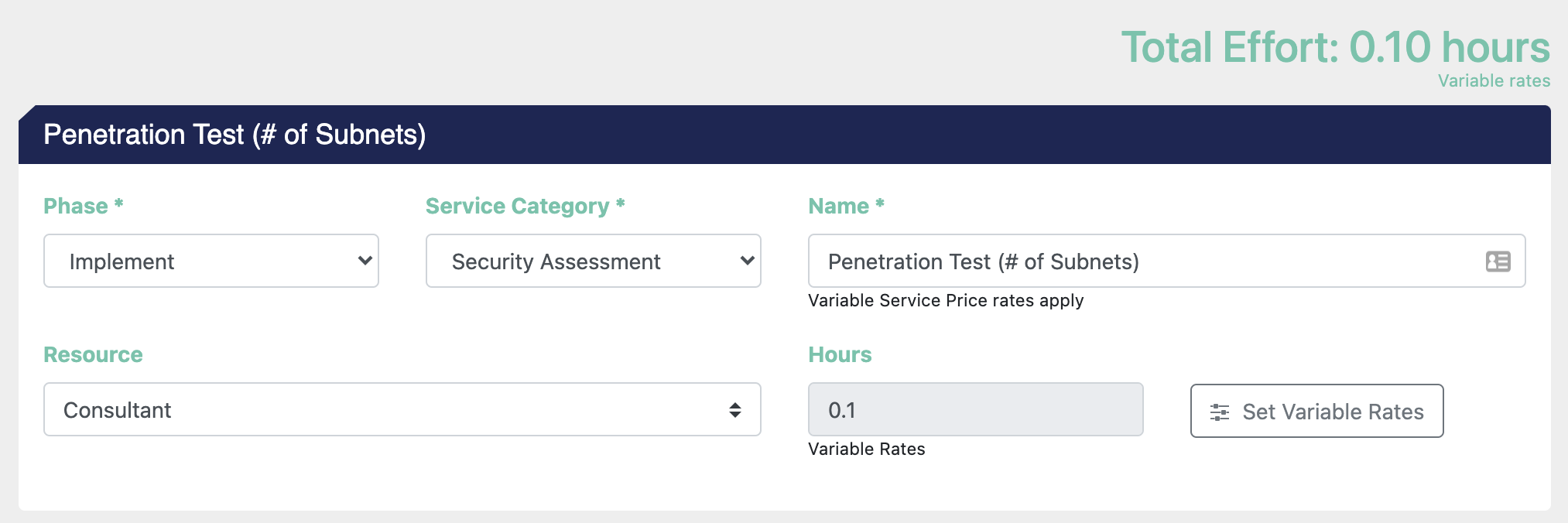• Note that the Total Effort amount in the top right and the Hours field let you know that Variable Rates were used to calculate effort.

• The note under the Name of the service indicates that Service Price rates apply.

In the Project...

• You can add a Service or Product that contains a variable rate to a project like any other element.

• Once added, the quantity will update the effort/pricing information as directed by the variable rate.

Variable Rates are a powerful feature that allows you even greater control of your project pricing in ScopeStack!Did this answer your question?